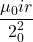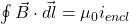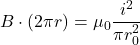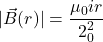## Find the magnitude of the magnetic field ∣∣B⃗ (r)∣∣ inside the cylindrical resistor, where r is the distance from the axis of the cylinder,

Question

Find the magnitude of the magnetic field ∣∣B⃗ (r)∣∣ inside the cylindrical resistor, where r is the distance from the axis of the cylinder, in terms of i, r, r0, l, and other given variables. You will also need π and μ0. Ignore fringing effects at the ends of the cylinder.

in progress 0
6 months 2021-07-30T07:46:06+00:00 1 Answers 176 views 0

The magnetic field inside the cylindrical resistor isExplanation:

Given that,

Distance from the axis of the cylinder = r

We need to calculate the magnetic field inside the cylindrical resistor

Using formula of magnetic fieldHence, The magnetic field inside the cylindrical resistor is